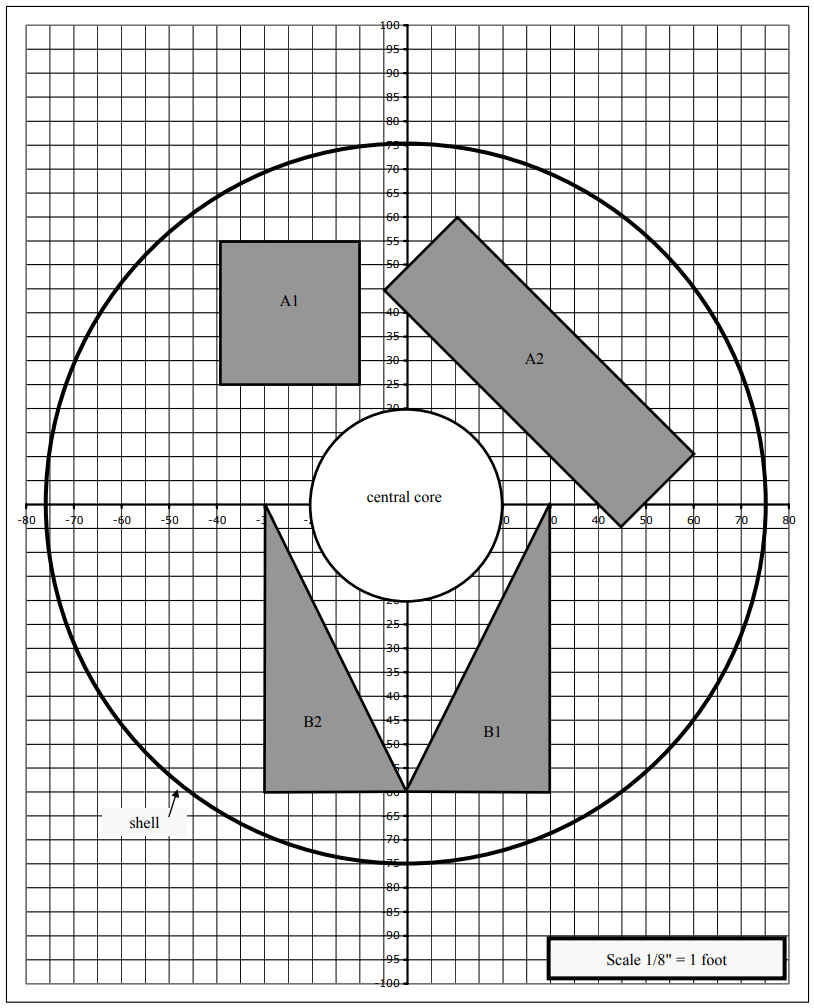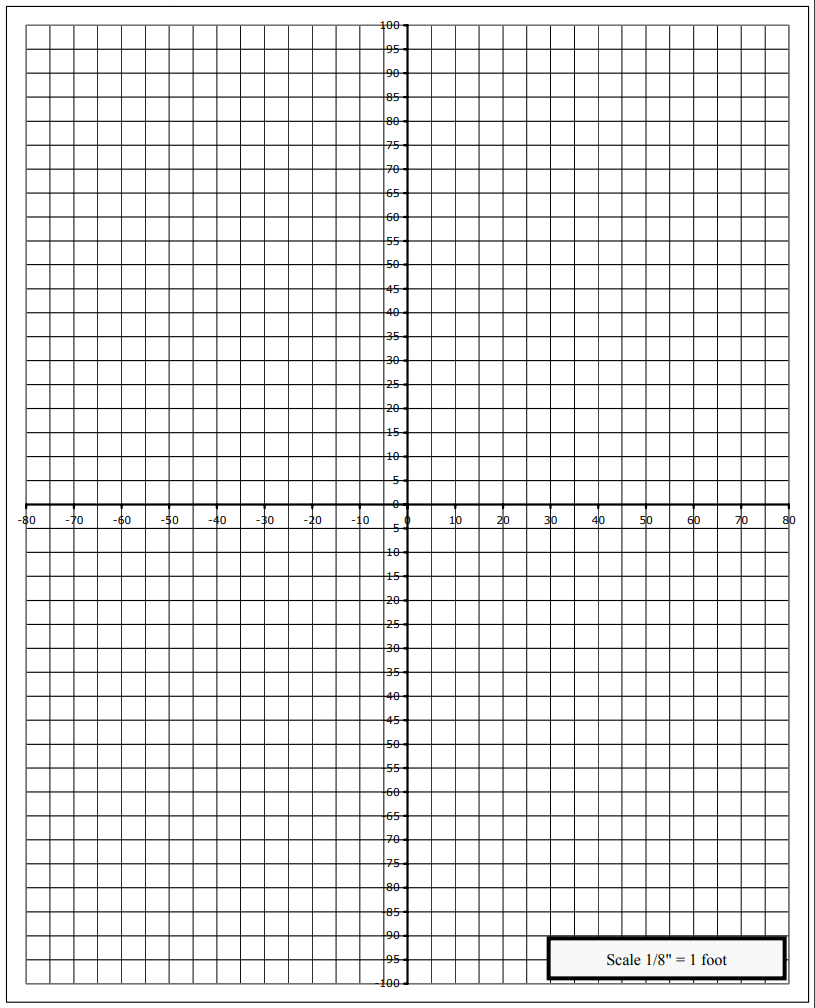Subject:
Mathematics

Topics: Algebra
Common Core State Standard: F-IF.5,
Concepts:

• Virtual

Knowledge and Skills:

• Can plot a linear function within specified limitations of x or y values.
• Can plot a circle given the equation

Lesson:

Procedure: Prepare for presentation the Futures Channel movie, Designing and Engineering Rockets.

Tell students that they will be watching a movie about rockets, and that, as they watch, you want them to think about this question:

Why would a systems engineer need to understand algebra?

Play the movie, The Ares Launch Vehicles, all the way through. Accept and discuss some answers to the prompt, then distribute the handout and review its instructions. Students may do the task individually or with a partner.

The next page shows the results of graphing the given equations.### VirtualRocketry

One tool that is used by systems engineers is a virtual model: a model of a system that exists in a computer, rather than in the real world.

In a virtual model, the parts of the system are all represented by mathematical equations in the computer. This activity will help you to understand how that works, as you create a virtual model of a cross-section of parts of a system that might exist in the second stage of a rocket.

The equations below represent parts of the system. Your task is to graph each equation in a Cartesian coordinate system using the included graph, then answer the questions below.

Central Core:

x2 + y2= 400

Part A1:

y = 55 for domain −40 ≤ x ≤ −10

y = 25 for domain −40 ≤ x ≤ −10

x = −10 for range 25 ≤ y ≤ 55

y = −40 for domain 25 ≤ y ≤ 55

Part A2:

y = −x + 70 for 10 ≤ x ≤ 60

y = −x + 40 for -5 ≤ x ≤ 45

y = x −40 for 45 ≤ x ≤ 60

y = x + 50 for -5 ≤ x ≤10

Part B2:

y = −2x − 60 for -30 ≤ x ≤ 0

y = −60 for -30 ≤ x ≤ 0

x = −30 for – 60 ≤ y ≤ 0

Shell:

x2 + y2= 5625

Questions:

1. Parts A1, A2, B1, and B2 should be separated by at least 2 feet. Are they?

2. All parts are to be separated from the central core by at least 3 feet. Are they?

3. All parts are to be separated from the shell by at least 4 feet. Are they?

4. What total square footage of cross-sectional area is occupied by parts A1, A2, B1 and B2?